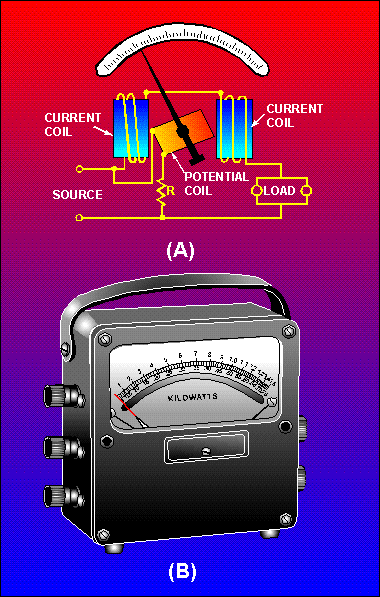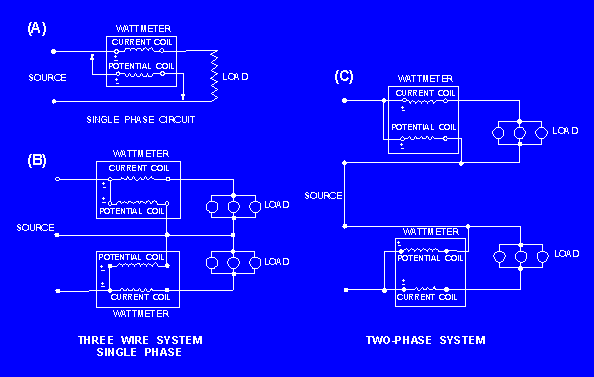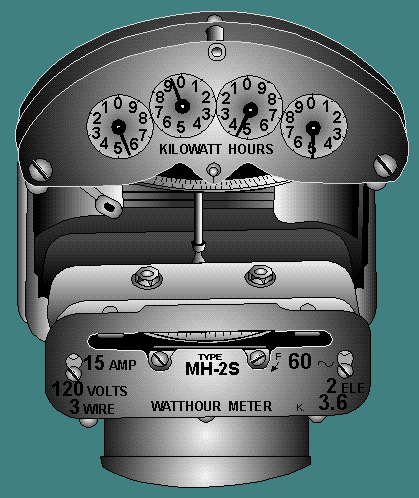Custom SearchWATTMETER Electric power is measured by means of a wattmeter. This instrument is of the electrodynamic type. It consists of a pair of fixed coils, known as current coils, and a movable coil known as the potential coil. (See fig. 1-44.) The fixed coils are made up of a few turns of a comparatively large conductor. The potential coil consists of many turns of fine wire. It is mounted on a shaft, carried in jeweled bearings, so that it may turn inside the stationary coils. The movable coil carries a needle which moves over a suitably marked scale. Spiral coil springs hold the needle to a zero position. Figure 1-44. - A simplified electrodynamic wattmeter circuit.The current coil (stationary coil) of the wattmeter is connected in series with the circuit (load), and the potential coil (movable coil) is connected across the line. When line current flows through the current coil of a wattmeter, a field is set up around the coil. The strength of this field is proportional to the line current and in phase with it. The potential coil of the wattmeter generally has a high-resistance resistor connected in series with it. This is for the purpose of making the potential-coil circuit of the meter as purely resistive as possible. As a result, current in the potential circuit is practically in phase with line voltage. Therefore, when voltage is applied to the potential circuit, current is proportional to and in phase with the line voltage. The actuating force of a wattmeter comes from the field of its current coil and the field of its potential coil. The force acting on the movable coil at any instant (tending to turn it) is proportional to the instantaneous values of line current and voltage. The wattmeter consists of two circuits, either of which will be damaged if too much current is passed through them. This fact is to be especially emphasized in the case of wattmeters, because the reading of the instrument does not serve to tell the user that the coils are being overheated. If an ammeter or voltmeter is overloaded, the pointer will be indicating beyond the upper limit of its scale. In the wattmeter, both the current and potential circuits may be carrying such an overload that their insulation is burning, and yet the pointer may be only part way up the scale. This is because the position of the pointer depends upon the power factor of the circuit as well as upon the voltage and current. Thus, a low power-factor circuit will give a very low reading on the wattmeter even when the current and potential circuits are loaded to the maximum safe limit. This safe rating is generally given on the face of the instrument. A wattmeter is always distinctly rated, not in watts but in volts and amperes. Figure 1-45 shows the proper way to connect a wattmeter in various circuits. Figure 1-45. - A wattmeter connected in various circuits. TWO-PHASE SYSTEMWATT-HOUR METER The watt-hour meter is an instrument for measuring energy. Since energy is the product of power and time, the watt-hour meter must take into consideration both of these factors. In principle, the watt-hour meter is a small motor whose instantaneous speed is proportional to the POWER passing through it. The total revolutions in a given time are proportional to the total ENERGY, or watt-hours, consumed during that time. The following directions should be followed when reading the dials of a watt-hour meter. The meter, in this case, is a four-dial type. The pointer on the right-hand dial (fig. 1-46) registers 1 kilowatt-hour, or 1,000 watt-hours, for each division of the dial. A complete revolution of the hand on this dial will move the hand of the second dial one division and register 10 kilowatt-hours, or 10,000 watt-hours. A complete revolution of the hand of the second dial will move the third hand one division and register 100 kilowatt-hours or 100,000 watt-hours, and so on. Figure 1-46. - Watt-hour meter.Accordingly, you must read the hands from left to right, and add three zeros to the reading of the lowest dial to obtain the reading of the meter in watt-hours. The dial hands should always be read as indicating the figure which they have LAST PASSED, and not the one they are approaching. Q.60 Why would you use a hook-on voltameter instead of a multimeter?Q.61 What electrical quantity is measured by a wattmeter?Q.62 What electrical quantity is measured by a watt-hour meter?Q.63 What is the quantity shown on the watt-hour meter in figure 1-46?Integrated Publishing, Inc. - A (SDVOSB) Service Disabled Veteran Owned Small Business